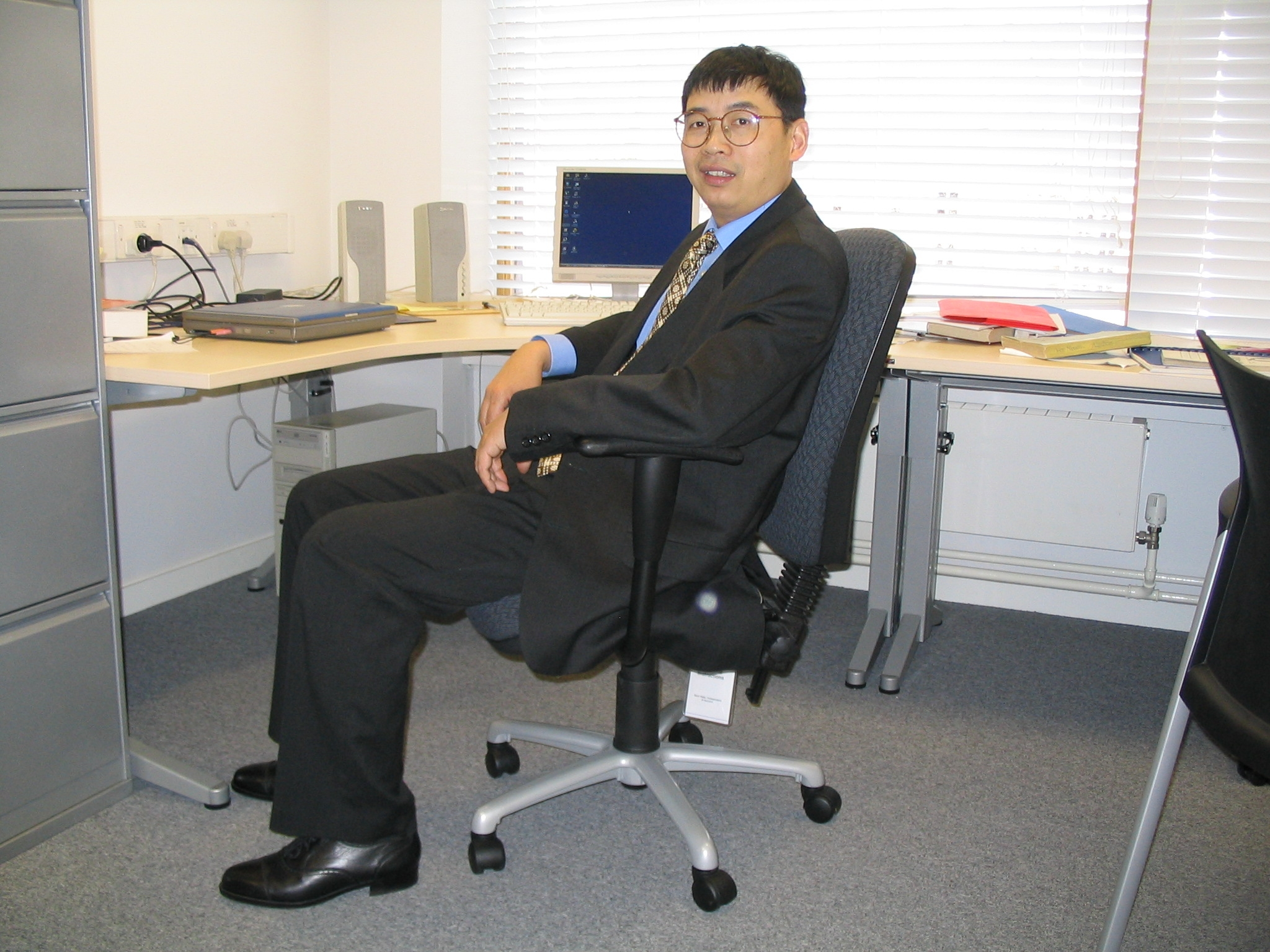Dr. Zhongmin QIANStochastic Analysis Group at Oxford SAG

Me

I am a University Lecturer in the Mathematical Institute, Oxford, and Fellow at Exeter College

Mailing Address: Mathematical Institute, University of Oxford, 24 - 29 St Giles', Oxford OX1 3LB, UK

Office: Math Institute S7

Tel: (01865) 273 563 (Institute),  (01865) 279 643 (College)

Email: qianz@maths.ox.ac.uk

Before joining Oxford I held positions

1. Royal Society Visiting Fellow at The University of Warwick (93-94)

2. Research Associate, and Lecturer at Imperial College (94-96, 98-99)

3. Visiting Fellow at l'Universite Paul-Sabatier, France (96-97)

4. Fellow at CNRS, France (since 1999 -- )

5. Visiting Professor, Cornell University, New York (2001-02)

6. Visiting Professor, Northwestern University, USA (2004)

Research Interests

I specialise in two different research areas: stochastic analysis, and non-linear partial differential equations coming from geometry.

Stochastic Analysis: Nowadays it is difficult to describe broad areas that the research in Stochastic Analysis covers. I am interested in mathematical models for random phenomena, called stochastic processes. A typical example of such processes, that has rich mathematics, is the well-known model for random movements observed by R. Brown, Brownian motion (also called Wiener process). A century ago, a French mathematician L. Bachelier in his thesis studied the mathematical model for stock markets via Brownian motion, and his ideas had been enforced over the past years, and had led to the famous Black-Scholes model for option pricing. My own research in recent years concentrate on

1. The theory of rough paths and its applications in stochastic analysis. The theory of rough paths was founded by Professor T. Lyons . A good account about this theory may be found in the book by T. Lyons and me, Rough Paths and System Control, published by Oxford University Press in 2002. One of long term goals of my research is to establish a theory of non-linear analysis for Wiener functionals, which the analysis of rough paths seems a right tool for the study of solutions to stochastic differential equations. Among my recent works, I, in collaboration with Dr Coutin (in Toulouse), Professor M. Ledoux and Professor T. Lyons, developed a stochastic calculus for stochastic processes with long-time memory, and for Wiener processes on Banach spaces. One can expect major applications of rough path theory in probability theory, geometric analysis on loop and path spaces, and in financial mathematics to come in the near future.

2. Analytic methods in probability theory. The semi-group technique has been an important tool in mathematics. In stochastic analysis, the standard theory of analytic semi-groups has been united with the Markov property which has produced a big industry in the past few years. The main problem is to relate asymptotic behaviours of random dynamic systems in terms of energy-entropy type inequalities (e.g. Poincare's inequality, Sobolev's inequality, isoperimetric inequality, Log-Sobolev inequality etc.), and how to devise energy-entropy type inequalities in terms of local properties of  dynamic systems. I am interested in random systems that can be described by Markov semi-groups. The local quantity I like very much is the curvature operator associated with the infinitesimal generator of a Markov semi-group. The curvature operator is a generalisation of the Ricci curvature in Riemannian geometry, which can be used in an efficient way to handle entropy-energy inequalities.

3. Statistics for Diffusion Processes. Most practical models in applications are diffusion models, models which are described by Ito's stochastic (partial) differential equations (SDE, or SPDE). In many questions, for example in various models for financial markets, these SDE or SPDE contain a number of unknown parameters for which we would like to determine or estimate. These practical questions lead to many interesting and in many instances quite difficult mathematical problems. One of such problems I tackled in last a couple of years was to determine extremal  probabilities for simple diffusions of Brownian motion with drifts, and I found explicit formulas in one dimensional case. Many open problems in this area are good Ph.D. thesis topics for those who may be interested in stochastic analysis and its applications, especially in financial and social studies.

Geometric Analysis. I find myself great fun with the Ricci curvature and its complex companion the first Chern class determined by a complex structure. I am mainly interested in analysis aspects of the Ricci curvature and complex structures, in particular, those (non-linear) partial differential equations (PDE) related to the Ricci curvature and the first Chern class.

1. Harnack's inequality.

2. 1-dimensional models for Riemannian spaces.

3. Ricci flow. In the past few years, there are exciting and major progresses in the study of Ricci flows and its application in geometry and topology, especially the substantial contributions made by G. Perelman in a series of papers. For some aspects about Ricci flows, an excellent book by B, Chow and D. Knopf (The Ricci Flow: An Introduction, AMS 2004) is available.

Ricci Flow and Perelman's Papers

I will post here some notes on Perelman's papers.

Recent preprints

1. Ricci flow on a 3-manifold with positive scalar curvature I. PDF file, a revised version is going to appear in Bull Math France.

2. Volume comparison theorems without Jacobi fields. PDF file

3. An estimate for the vorticity of the Navier-Stokes equation, to appear in Comptes rendus - Mathematique.

Invited seminar talks (2004)

1. PDE seminar at Northwestern University, Evanston, USA.

2. Stochastic Analysis seminar (April 2004), University of Illinois at Urbana-Champaign.

3. Geometric Analysis seminar (April 2004), Princeton University.

4. Non-linear Analysis seminar (Sept. 2004), Fudan University, China.

5. Stochastic Analysis seminar (Oct. 2004), University of Oxford.

Conference and Seminar talks (2005)

1. Ricci flow on manifolds, UK-Japan Winter School, 9-12 January 2005.

2. Ricci flow on manifolds with positive scalar curvature, Geometry and analysis seminars, Oxford, 24 January 2005.

3. Several talks at Osaka and Sendai, Japan, August, 2005.

Teaching (Michaelmas term 2005)

I will lecture ''Stochastic Differential Equations'' (Tuesdays at 4 PM and Fridays at 3 PM). Here is the link to the course homepage

SDE Michaelmas Term 2005

BMC2007